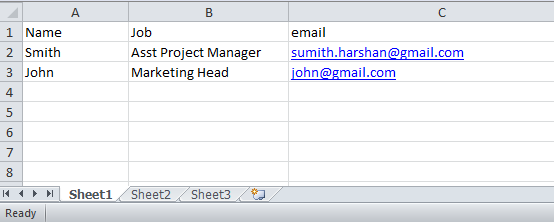# Read Excel file and save details to database using PHP

How can you read and display excel file using php and save that details to the database? You can use this simple php class to do this.That is read row by row while reading column by column.While reading like this it gets each cells details.Look at this example that is simply describes this process and you can understand this better.

Here is the sample.xls file format.## How to read excel file using php

```

Sheet 1:

read('sample.xls'); // set the excel file name here
\$x=1;
while(\$x<=\$excel->sheets['numRows']) { // reading row by row
echo "\t\n";
\$y=1;
while(\$y<=\$excel->sheets['numCols']) {// reading column by column
\$cell = isset(\$excel->sheets['cells'][\$x][\$y]) ? \$excel->sheets['cells'][\$x][\$y] : '';
echo "\t\t\n";  // get each cells values
\$y++;
}
echo "\t\n";
\$x++;
}
?>
\$cell

```

Note:
This is reading sheet1 details.If you want to read sheet 2 details, then change the sheets to sheets in both while loops.
Example:
`while(\$x<=\$excel->sheets['numRows']) { `
Remember “sheets” is the array.Array begin from 0.You already know about it. 😀
So,
Sheet1 -> sheets
Sheet2 -> sheets
Sheet3 -> sheets

## How to read and save details to the database using php

Table structure

```DROP TABLE IF EXISTS `users_details`;
CREATE TABLE `users_details` (
`id` int(11) NOT NULL auto_increment,
`name` varchar(255) default NULL,
`job` varchar(255) default NULL,
`email` varchar(255) default NULL,
PRIMARY KEY  (`id`)
) ENGINE=InnoDB AUTO_INCREMENT=7 DEFAULT CHARSET=latin1;
```
```

Save Excel file details to the database

\$x=2;
while(\$x<=\$excel->sheets['numRows']) {
\$name = isset(\$excel->sheets['cells'][\$x]) ? \$excel->sheets['cells'][\$x] : '';
\$job = isset(\$excel->sheets['cells'][\$x]) ? \$excel->sheets['cells'][\$x] : '';
\$email = isset(\$excel->sheets['cells'][\$x]) ? \$excel->sheets['cells'][\$x] : '';
// Save details
\$sql_insert="INSERT INTO users_details (id,name,job,email) VALUES ('','\$name','\$job','\$email')";
\$result_insert = mysql_query(\$sql_insert) or die(mysql_error());
\$x++;
}
?>

```

That’s only.